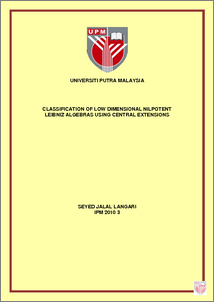# Classification Of Low Dimensional Nilpotent Leibniz Algebras Using Central Extensions

## Citation

Langari, Seyed Jalal (2010) Classification Of Low Dimensional Nilpotent Leibniz Algebras Using Central Extensions. PhD thesis, Universiti Putra Malaysia.

## Abstract

This thesis is concerned with the classification of low dimensional nilpotent Leibniz algebras by central extensions over complex numbers. Leibniz algebras introduced by J.-L. Loday (1993) are non-antisymmetric generalizations of Lie algebras. There is a cohomology theory for these algebraic objects whose properties are similar to those of the classical Chevalley-Eilenberg cohomology theory for Lie algebras. The central extensions of Lie algebras play a central role in the classification theory of Lie algebras. We know that if a Leibniz algebra L satisfies the additional identity [x; x] = 0; x E L, then the Leibniz identity is equivalent to the Jacobi identity [[x; y]; z] + [[y; z]; x] + [[z; x]; y] = 0 8x; y; z E L: Hence, Lie algebras are particular cases of Leibniz algebras.In 1978 Skjelbred and Sund reduced the classification of nilpotent Lie algebras in a given dimension to the study of orbits under the action of a group on the space of second degree cohomology of a smaller Lie algebra with coefficients in a trivial module. The main purpose of this thesis is to establish elementary properties of central extensions of nilpotent Leibniz algebras and apply the Skjelbred-Sund's method to classify them in low dimensional cases. A complete classification of three and four dimensional nilpotent Leibniz algebras is provided in chapters 3 and 4. In particular, Leibniz central extensions of Heisenberg algebras Hn is provided in chapter 4. Chapter 5 concerns with application of the Skjelbred and Sund's method to the classification of filiform Leibniz algebras in dimension 5. Chapter 6 contains the conclusion and some proposed future directions.Preview
PDF
IPM_2010_3A.pdfView Item